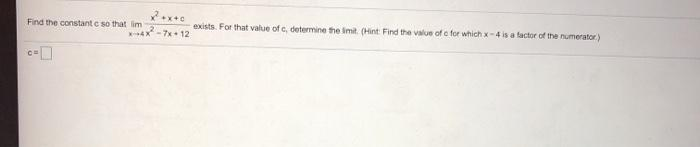### Create an Account

Already have account?

### Forgot Your Password ?

Home / Questions / Find the constant c so that im exists. For that value of c, determine the imit (Hint Find ...

# Find the constant c so that im exists. For that value of c, determine the imit (Hint Find the value of for which X-4 is a factor of the numerator) X-7x+12 CE

Find the constant c so that im exists. For that value of c, determine the imit (Hint Find the value of for which X-4 is a factor of the numerator) X-7x+12 CEApr 19 2021 View more View Less

#### Answer (Solved)Subscribe To Get Solution